# NCERT Solutions for Class 6 Maths Fraction Exercise 7.5 and 7.6

In this page we have Ncert Solutions for Class 6 Maths Fraction Exercise 7.5 and 7.6. Hope you like them and do not forget to like , social share and comment at the end of the page.

# Exercise 7.5

Question 1
Write these fractions appropriately as additions or subtractions:
(a)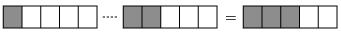(b)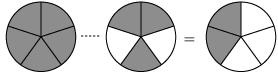(c)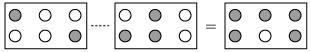a) First fraction 1/5 Second Fraction 2/5 Third Fraction 3/5 1/5 + 2/5= 3/5 Addition b) First fraction =5/5 Second fraction =3/5 Third fraction =2/5 1- 3/5= 2/5 Subtraction c) First fraction= 2/6 Second Fraction=3/6 Third Fraction 5/6 2/6+ 3/6= 5/6 Addition
Question 2
Solve:
(a)$\frac {1}{18} + \frac {1}{18}$
(b)$\frac {8}{15} + \frac {3}{15}$
(c)$\frac {7}{7} - \frac {5}{7}$
(d)$\frac {1}{22} + \frac {21}{22}$
(e)$\frac {12}{15} - \frac {7}{15}$
(f)$\frac {5}{8} + \frac {3}{8}$
(g)$1 - \frac {2}{3}$
(h)$\frac {1}{4} + \frac {0}{4}$
(i)$3 - \frac {12}{5}$
If the denominator is same, we can add and subtract the numerator
 a) $\frac {1}{18} + \frac {1}{18}$ 2/18=1/9 b) $\frac {8}{15} + \frac {3}{15}$ 11/15 c) $\frac {7}{7} - \frac {5}{7}$ 2/7 d) $\frac {1}{22} + \frac {21}{22}$ 22/22=1 e) $\frac {12}{15} - \frac {7}{15}$ 5/15=1/3 f) $\frac {5}{8} + \frac {3}{8}$ 8/8=1 g) $1 - \frac {2}{3}$ 1 can be written as 3/3 So 1/3 h) $\frac {1}{4} + \frac {0}{4}$ ¼ i) $3 - \frac {12}{5}$ 15/5 – 12/5= 3/5
Question 3
Shubham painted 2/3 of the wall space in his room. His sister Madhavi helped and painted 1/3 of the wall space. How much did they paint together?
Space painted by them together = Space painted by Shubham + Space painted by Madhavi
= 2/3 + 1/3= 3/3=1
So, they painted the entire wall.
Question 4
Fill in the missing fractions.
(a)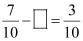(b)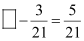(c)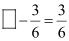(d)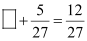a) Missing fraction will be 7/10 -3/10 = 4/10
b) Missing fraction will be 5/21 + 3/21 = 8/21
c) 1
d) 7/27
Question 5
Javed was given 5/7 of a basket of oranges. What fraction of oranges was left in the basket?
Fraction of oranges given to Javed = 5/7

Fraction of oranges left in the basket =
1 -5/7 =2/7

# Exercise 7.6

Question 1
Solve
(a)$\frac {2}{3} + \frac {1}{7}$
(b)$\frac {3}{10} + \frac {7}{15}$
(c)$\frac {4}{9} + \frac {2}{7}$
(d)$\frac {5}{7} + \frac {1}{3}$
(e)$\frac {2}{5} + \frac {1}{6}$
(f)$\frac {4}{5} + \frac {2}{3}$
(g)$\frac {3}{4} - \frac {1}{3}$
(h)$\frac {5}{6} - \frac {1}{3}$
(i)$\frac {2}{3} + \frac {3}{4} + \frac {1}{2}$
(j)$\frac {1}{2} + \frac {1}{3} + \frac {1}{6}$ (k)$1\frac {1}{3} + 3\frac {2}{3}$
(l)$4\frac {2}{3} + 3\frac {1}{4}$
(m)$\frac {16}{5} - \frac {7}{5}$
(n)$\frac {4}{3} - \frac {1}{2}$
In these questions, the denominator is different, so we cannot simply add the numerator. We have to first find the LCM of the denominators and then convert each fraction into that form and then do numerators addition or subtraction
a) LCM of 3 and 7 =21
b) LCM of 10 and 15 is 30
so answer is 23/30
c) LCM of 9 and 7 is 63
so answer is 46/63
d) LCM of 7 and 3 is 21
so answer is 22/21
e) LCM of 5 and 6 =30
so answer is 17/30
f) LCM of 5 and 3 =15
so answer is 22/15
g) LCM of 4 and 3 =12
h) LCM of6 and 3 =6
i) LCM of 2,4,3 =12
j) LCM 2,3,6=6
k) Changing mixed fraction into improper fraction
l) Changing mixed fraction into improper fraction
LCM of 3 and 4 =12
m) 9/5
n) 5/6
Question 2
Saria bought 2/5 metre of ribbon and Lalita ¾ metre of ribbon. What is the total length of the ribbon they bought?
Length of ribbon Saria bought = 2/5 meter
Length of ribbon Lalita bought = ¾ meter
Total length of ribbon = 2/5 + 3/4
They bought 23/20 meters of ribbon together
Question 3
Naina was given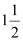piece of cake and Najma was given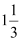piece of cake. Find the total amount of cake was given to both of them.
Total amount of cake both have
=+This is mixed fraction, converting into improper fraction
= 3/2 + 4/3
=17/6
So they total have 17/6 pieces of cake
Question 4
Fill in the boxes:
(a)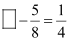(b)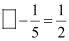(c)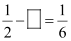Question 5
Complete the addition-subtraction box.
(a)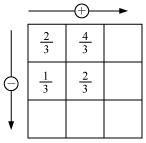(b)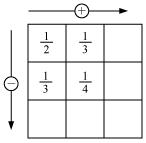Question 6
A piece of wire 7/8-metre-long broke into two pieces. One piece was ¼ metre long. How long is the other piece?
Length of the piece of wire is =7/8 meter
Length of one broken piece is =1/4 meter
Length of the other broken piece = total length of the wire – length of one broken piece
=7/8 – ¼=   5/8 m
Length of the piece of wire is 5/8 meter
Question 7
Nandini’s house is 9/10 km from her school. She walked some distance and then took a bus for ½ km to reach the school. How far did she walk?
Distance from Nandini’s house to school =9/10 km
Distance covered by bus = ½ km
Distance she walked = distance from house to school – distance she covered by bus.

=9/10  - ½ = 4/10 = 2/5 km

Distance walked by Nandhini = 2/5 km
Question 8
Asha and Samuel have bookshelves of the same size partly filled with books.
Asha’s shelf is 5/6th full and Samuel’s shelf is  2/5 th full. Whose bookshelf is more full? By what fraction?
Fraction of books in Asha’s shelf =5/6
Fraction of books in Samuel’s shelf =2/5
We compare the fractions to find whose shelf is more full.
Since these are unlike fraction, we need to first find the LCM of 5 and 6 which is 30
5/6 = 25/30
2/5= 12/30
So 5/6 > 2/5
So, Asha’s shelf has more books and is more full.
Fraction by which Asha bookshelf is more full
=5/6 -2/5 = 13/30

Asha’s shelf is more full and by 13/30
Question 9
Jaidev takes $2 \frac {1}{5}$ minutes to walk across the school ground. Rahul takes 7/4 minutes to do the same. Who takes less time and by what fraction?
Time taken by Jaidev to walk across the ground =11/5 min
Time taken by Rahul to walk across the ground =7/4 min
We compare the fractions to find who takes is less time
Since these are unlike fraction, we need to first find the LCM of 5 and 4 which is 20
11/5 = 44/20
7/4= 35/20
Clearly 7/4 < 11/5
So, Rahul takes lesser time than Jaidev.
Difference =11/5 – 7/4 = 9/20

So, Rahul takes lesser time than Jaidev, by 9/20

### Practice Question

Question 1 What is $\frac {1}{2} + \frac {3}{4}$ ?
A)$\frac {5}{4}$
B)$\frac {1}{4}$
C)$1$
D)$\frac {4}{5}$
Question 2 Pinhole camera produces an ?
A)An erect and small image
B)an Inverted and small image
C)An inverted and enlarged image
D)None of the above